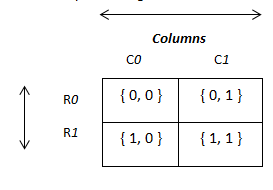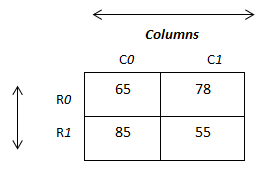• C supports multi dimension arrays. The simplest form of the multi-dimension array is the two dimensional array.
• An array having two subscripts is known as two-dimensional array.
• Two-dimension array is a collection of rows and columns so it can be represented by the matrix in mathematics form.
• It can be represented as a series or collection of one-dimension array. It allows storing data in tabular format.
• The matrix elements can be represented by Vij where ‘i’ value indicates the row number and ‘j’ value indicated the column number.

### Declaration of Two-Dimension Array:

• We can declare two-dimension array using the following syntax:
``<data-type> arr-var-name [row size] [column size] ;``
• Here, <row size> and <column size> specifies total number of rows and columns respectively.
• The following example declares a two- dimensional integer array with 2 rows and 2 columns (2×2).
`int x;`
• Memory representation of array x can be given as follow:• Here intersection of rows and columns creates a cell and each cell location can be identified by using its row index and column index.
• For example, the location of the first element will be 0th row and 0th column ({0, 0}), for second element 0th row and 1st column ( { 0, 1 } ), 1st row and 0th column for thirst element ( { 1, 0 } ) and 1st row and 1st column for fourth element ( { 1, 1 } ).

### Initialization of Two Dimension Array:

• Similar to one-dimensional array, Two-dimension array can also be initialized either statically or dynamically.

#### Static initialization:

• We can initialize the above x array variable using row and column index either individually or in a group as follow:
`x  = 65 ;`
• We can combine array declaration and initialization into a single statement as follow:
```int x  = {  { 65, 78 } ,
{ 85, 55 }
} ;```
• The above statement will declare array called x of integer types with the size of 2 rows and 2 columns.
• Notice that each row elements are represented in its own set of curly brackets and they are separated by comma.
• It will assign first value to 0th row and 0th column, second value to 0th row and 1st column and so on.
• Memory representation of array x can be given as follow:Note: If you want to initialize the all the array elements with the zero then you can do by:

`int x  = { 0 };`

#### AccessingTwo-Dimension Array Elements:

• We can access each array elements by using its row index as well as column index within square brackets along with array variable name.
• For example, to access the first element of the array x, you can use x , for second element x , and so on.
• The following example performs total of array elements of x by accessing them using their index.
` total = x   +  x    +   x    +   x  ;`

#### Dynamic initialization:

• We can also initialize array at run time. It is referred to as dynamically initialization.
• The following example uses for loop to initialize x array from user at run time.
• Recall that for initialization of single dimension array we used single loop as it contained only one dimension.
• Since two dimension array contains rows and columns we need two loops to access array elements: the outer for loop that keeps track of rows and inner for loop that keeps track of column inside the current row.
• The row loop will be executed as per the row size and column loop for the number of columns times as follow:
``` for ( i=0 ; i < 2 ;  i++ )  //   iterates over rows
{
for ( j=0 ; j < 2 ;  j++ ) // iterates over columns in current row
{
printf(“\n Enter x[%d][%d]:”, i,j ) ;
scanf( “ %d “ , &x [i][j] ) ;
}
}```
• The above for loop is executed for four times which is the size of our array x and reads four value from the user and each time counter variable gets incremented and based on its value it is assigned in array x i.e. first value will be stored to x (as initially i is 0 & j is also 0), second value will be stored to x  (as i = 0 and j=1) and so on.
• The following program demonstrates reading four values into array and displaying on the screen.
```/* Program to demonstrate the use of 2D-Array */

#include <stdio.h>

void main(void)
{
int marks;    /* this declares integer array of 3x2 */
int i, j;

clrscr();

/* read data from the keyboard  */
for ( i=0 ; i < 3 ;  i++ )  //   iterates over rows
{
for ( j=0 ; j < 2 ;  j++ ) // iterates over columns in current row
{
printf(“\n Enter x[%d][%d]:”, i,j ) ;
scanf( “ %d “ , &x [i][j] ) ;
}
}

/* display contents of marks */
for ( i=0 ; i < 2 ;  i++ )  //   iterates over rows
{
for ( j=0 ; j < 2 ;  j++ ) // iterates over columns in current row
{
printf(“\t %d “, x[i][j]) ;
}

printf( “\n” ) ;       //  prints new row on new line
}
}```
• In the case of a two -dimensional array, the following formula is used to compute the number of bytes of memory require storing it:

bytes  =  size  of  row   ×   size  of  column  ×   sizeof(base  type)

• For example, bytes required for the given integer array x with size of 3 rows and 5 columns will be computed as follow:
`int x ;`

total bytes = 3 (sizeof row ) X  5  (sizeof column ) X 2 ( since integer requires 2 bytes)

`total bytes = 30`